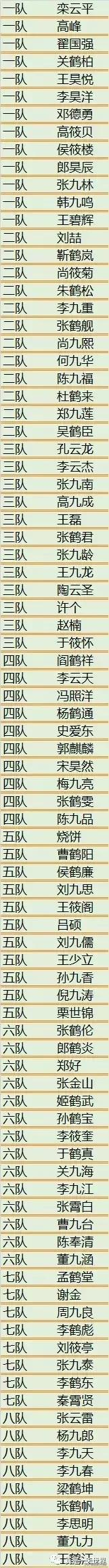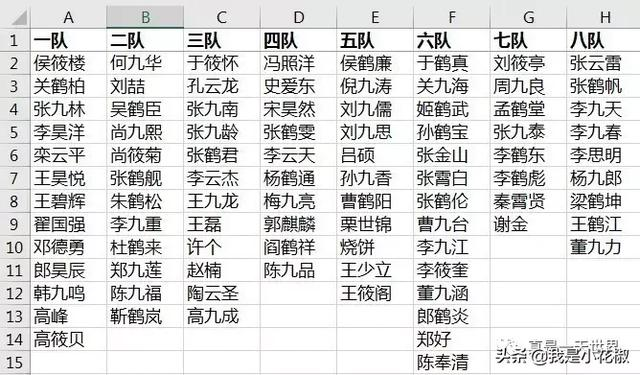1. 增加辅助列 C 和 D，公式分别如下：

=COUNTIF(A\$1:A1,A1)

=INDEX(A:A,SMALL(IF(\$C:\$C=1,ROW(\$A:\$A),4^8),ROW(1:1)))&""

• 这两个辅助列的目的是生成一个去重去空的队名列表
• D 列为数组公式，需要按 Ctrl Shift Enter 生效
• 具体公式释义请参见 去除Excel下拉菜单中的空值和重复值 和 Excel下拉菜单去空/去重（填坑贴）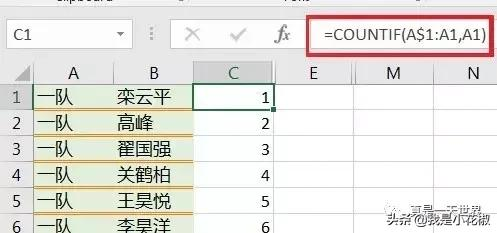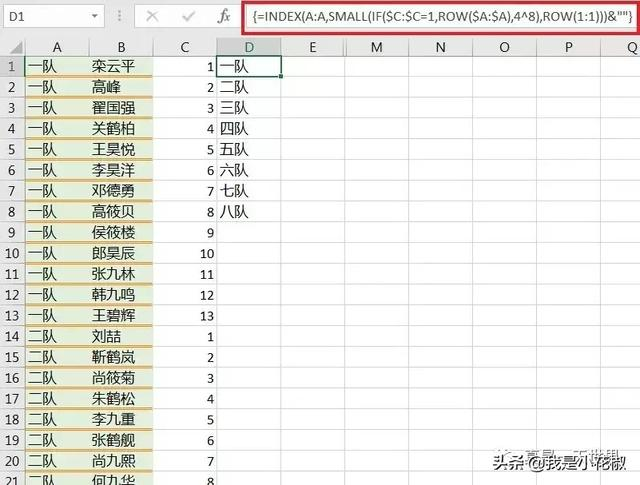2. 在 F 列输入如下公式，目的是把 D 列的竖表变为横表：

=INDIRECT("D"&COLUMN(A1))

• 关于这段 indirect 函数的释义，请参见 Excel indirect 函数(1) - 将一列数据排列成m行*n列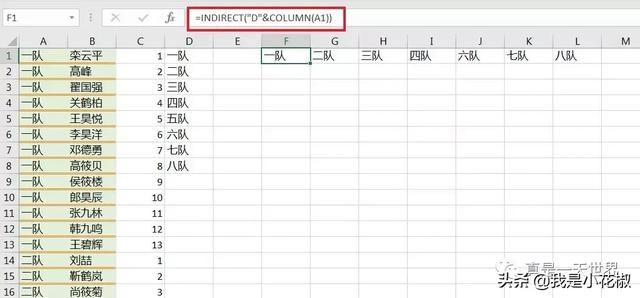3. 在 F2 单元格输入如下公式，按 Ctrl Shift Enter 生效，向下向右拖动公式，即可完成整张表格：

=INDEX(\$B:\$B,SMALL(IF(F\$1=\$A:\$A,ROW(\$B:\$B),4^8),ROW(1:1)))&""

• 表示当 A 列的队名与 F1 至 L1 相同时，按列读出队员名，否则返回空值
• 这是数组公式，需要按 Ctrl Shift Enter 生效
• 万能数组公式 index small if row 的释义请参见 去除Excel下拉菜单中的空值和重复值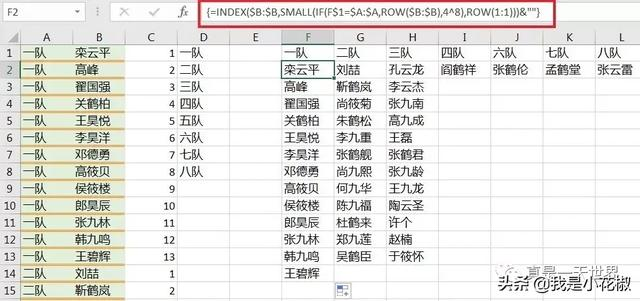想要从零系统学习Excel，查看本站上方“视频教程”获取最新秋叶Office三合一办公应用课程！